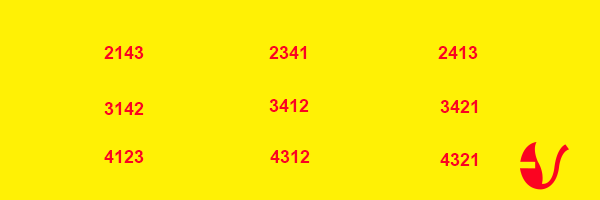# Concept of DERANGEMENTS Theorem

DERANGEMENTS

The word derangement in simple words means any change in the existing order of things. Mathematically, derangement refers to the permutation consisting of elements of a set in which the elements don’t exist in their respective usual positions.

We consider a simple example to understand this concept. Suppose that a teacher has given assignments to 4 groups. Now, obviously a group cannot grade or assess his own assignment and so the teacher wants the groups to grade each other’s assignment.

Our first concern is that in how many ways the teacher can provide the assignments to groups 1, 2, 3 and 4 the so that none of the group gets its own. In this case, the total possible number of permutations is 4! = 24. But, out of these there are only 9 derangements. The possible derangements are listed below:

In the remaining permutations except the above list, at least one group will receive its own assignment.Hence, derangements can basically be assumed to be the permutations in which the positions of the elements are altered. The only possible derangements of the set {1, 2, 3} are {2, 3, 1} and {3, 1, 2}. Another point to be noted is that derangements don’t contain cycles or permutations of length one.

On similar lines of the problem discussed above, another question that is asked on derangement is of envelopes. If we are asked the total number of ways of placing n letters in n already addressed envelopes, wherein every letter is addressed to a different person in such a way that none of the letter is placed in the correctly addressed envelope.

This is the most general question on the derangement principle that is often asked in the examinations. If ‘n’ particular items need to be arranged or organized in a row in such a manner that none of them acquires its usual or correct place is calculated using the formula:
$$n!\left( {1 - \frac{1}{{1!}} + \frac{1}{{2!}} - \frac{1}{{3!}} + \,.\,.\,.\,. + {{\left( { - 1} \right)}^n}\frac{1}{{n!}}} \right)$$        $$= n!\sum\nolimits_{r = 0}^n {} {\left( { - 1} \right)^r}\frac{1}{{r!}}$$

Illustration:
Supposing 4 letters are placed in 4 different envelopes. In how many ways can they be taken out from their original envelopes and distributed among the 4 different envelopes so that no letter remains in its original envelope?

Solution:
This is clearly a case of derangement. Using the formula for the number of derangements that are possible out of 4 letters in 4 envelopes, we get the number of ways as:
\eqalign{ & = 4!\left( {1 - 1 + \frac{1}{{2!}} - \frac{1}{{3!}} + \frac{1}{{4!}}} \right) \cr & = 24\left( {1 - 1 + \frac{1}{2} - \frac{1}{6} + \frac{1}{{24}}} \right) \cr & = 9 \cr}